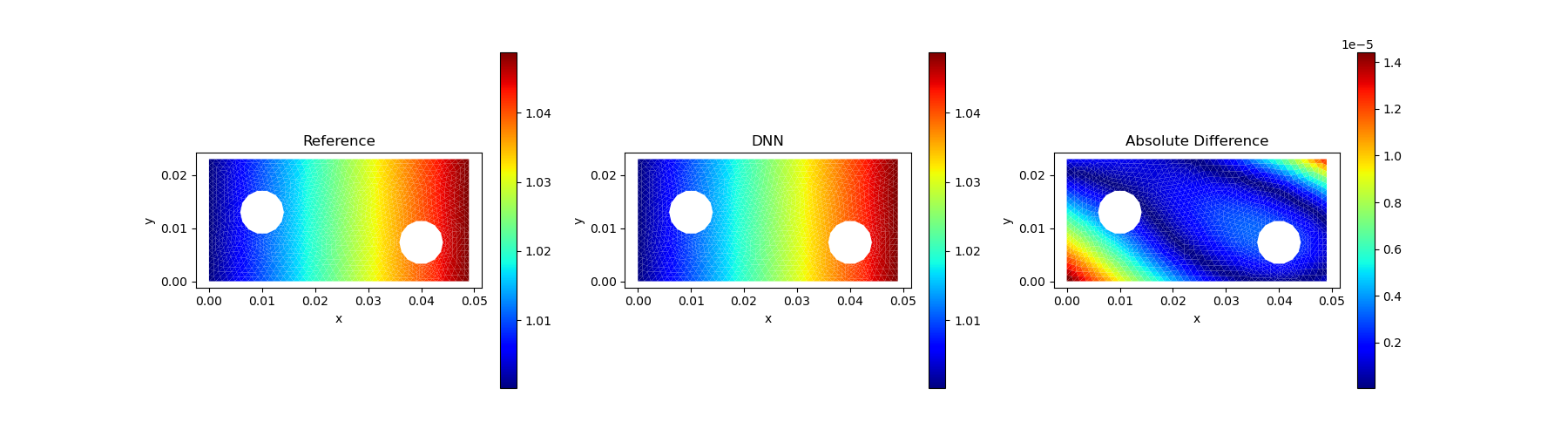Innovative, efficient, and computational-graph-based finite element simulator for inverse modeling
Author kailaix
Popularity
25 Stars
Updated Last
1 Year Ago
Started In
January 2020

AdFem is a finite element method open source library for inverse modeling in computational and mathematical engineering. It provides a set of reusable, flexible, and differentiable operators for building scalable and efficient simulators for partial differential equations.

AdFem is built on ADCME, an automatic differentiation library for computational and mathematical engineering. It was originally developed for prototyping inverse modeling algorithms using structured meshes but later evolved into a general and powerful tool with a scalable FEM backend MFEM.

`Ad` in AdFem stands for "automatic differentiation" or "adjoint".

As an example, we consider solving the Poisson's equation in AdFemHereThe weak form for the Poisson's equation is to solve a variational equationThe problem is easily translated in AdFem:

```using AdFem
using PyPlot

# forward computation
mmesh = Mesh(joinpath(PDATA, "twoholes_large.stl"))
xy = gauss_nodes(mmesh)
κ = @. sin(xy[:,1]) * (1+xy[:,2]^2) + 1.0
f = 1e5 * @. xy[:,1] + xy[:,2]
K = compute_fem_laplace_matrix1(κ, mmesh)
F = compute_fem_source_term1(f, mmesh)
bdnode = bcnode(mmesh)
K, F = impose_Dirichlet_boundary_conditions(K, F, bdnode, zeros(length(bdnode)))
sol = K\F```

The above code shows how to use a linear finite element space to approximate the state variable on a given mesh, define boundary conditions, and construct the linear system.

## Inverse Modeling

Most functions of AdFem, such as `compute_fem_laplace_matrix1`, `compute_fem_source_term1`, and `impose_Dirichlet_boundary_conditions`, are AD-capable, meaning that you can back-propagate gradients from their outputs to inputs. This enables you to conduct inverse modeling without writing extra substantial effort once the forward computation codes are implemented. AdFem constructs a static computational graph for finite element simulators: the computational graph is optimized before executation, and all computations are delegated to efficient C++ kernels.

Here we use a deep neural network to approximate κ(x) (`fc` is an ADCME function and stands for fully-connected):

```nn_κ = squeeze(fc(xy, [20,20,20,1])) + 1
K = compute_fem_laplace_matrix1(nn_κ, mmesh)
F = compute_fem_source_term1(f, mmesh)
bdnode = bcnode(mmesh)
K, F = impose_Dirichlet_boundary_conditions(K, F, bdnode, zeros(length(bdnode)))
nn_sol = K\F
loss = sum((nn_sol - sol)^2)

sess = Session(); init(sess)
BFGS!(sess, loss)```## Installation

AdFem is tested on Unix platform (Linux and Mac). To install the stable release:

```using Pkg

```using Pkg

To install with docker, here is the built docker image provided by @Ricahrd-Li, and please see docker install guide.

## Research

AdFem is an open-source package that accompanies ADCME.jl for solving inverse problems involving partial differential equations (PDEs). AdFem provides users a rich collection of operators, which users can use to quickly build finite element/volumn codes for forward computation. More importantly, these operators can back-propagate gradients, and therefore users can calculate the gradients using the ideas of adjoint methods and reverse-mode automatic differention (these two concepts overlap). The advanced physics constrained learning (PCL) approach enables users to back-propagate gradients through iterative and nonlinear solvers efficiently. AdFem offers a flexible interface for experienced researchers to develop their own operators.

Some related research works can be found here: